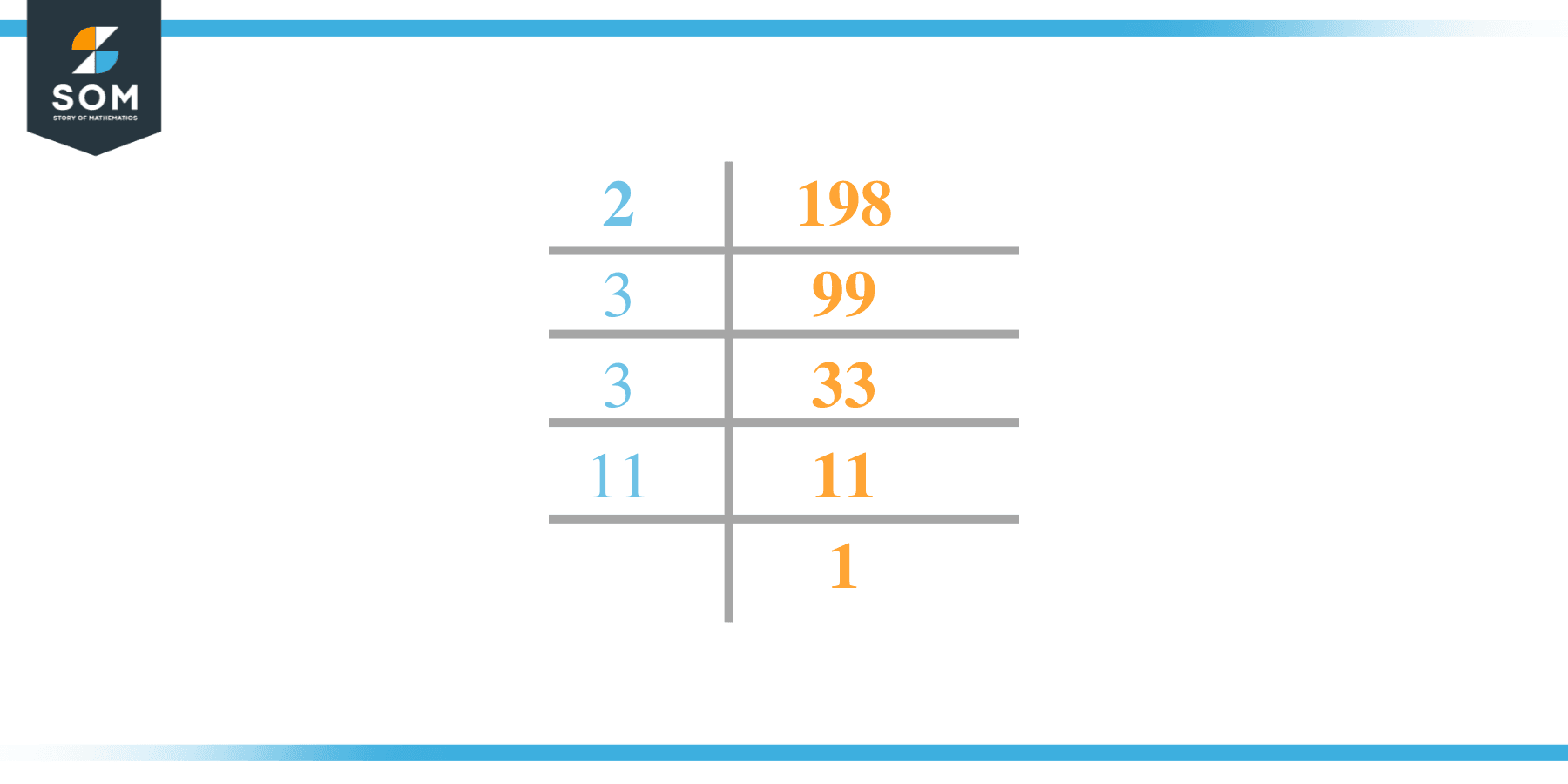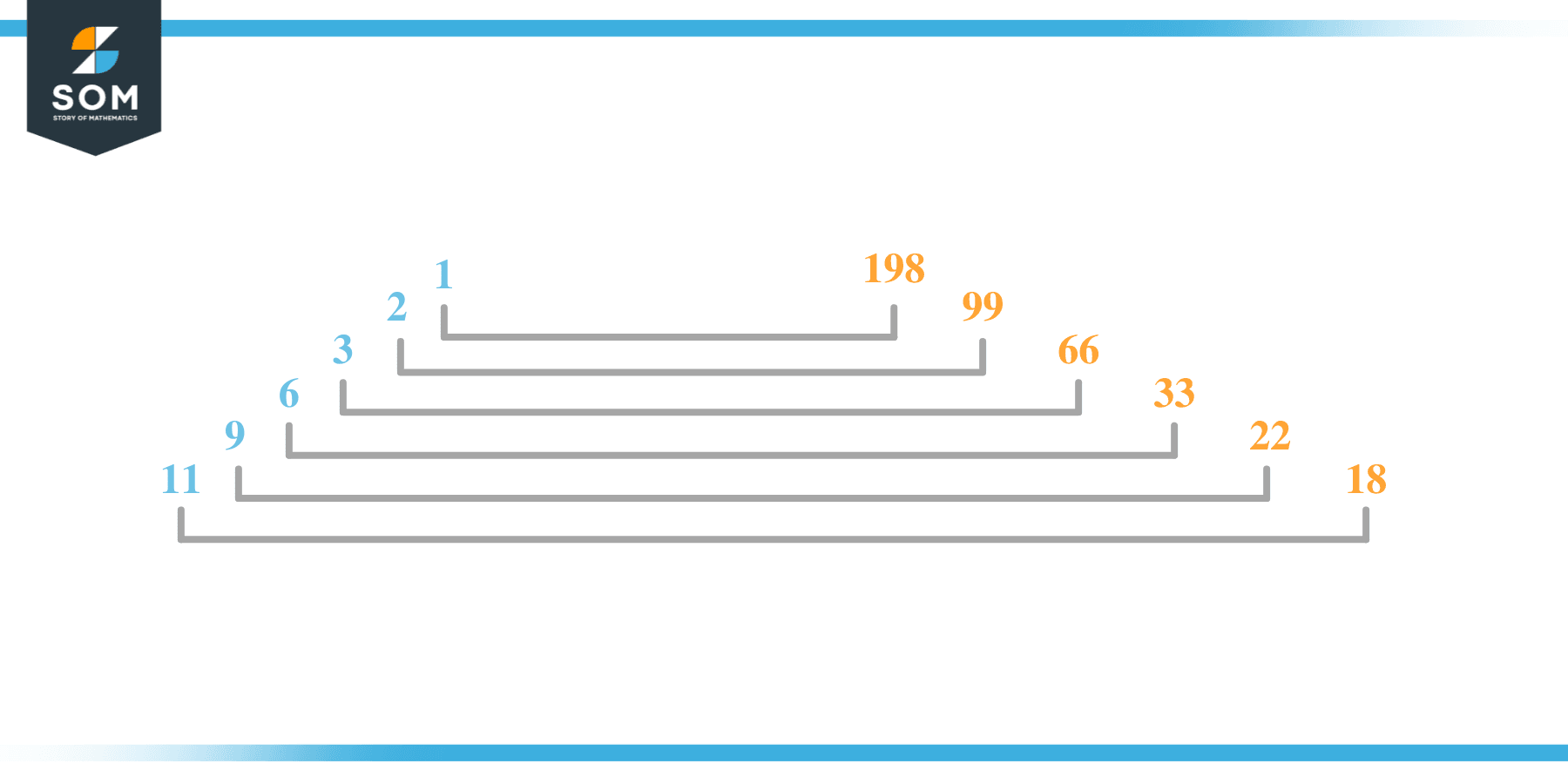# Factors of 198: Prime Factorization, Methods, and Examples

The factors of 198 are the numbers that leave zero as the remainder. Since 198 is an even composite number so it consists of multiple factors, including 2.These factors can be both positive and negative and can be found by two methods – prime factorization and the division method.

### Factors of 198

Here are the factors of number 198.

Factors of 198: 1, 2, 3, 6, 9, 11, 18, 22, 33, 66, 99, 198

### Negative Factors of 198

The negative factors of 198 are similar to its positive factors, just with a negative sign.

Negative Factors of 198: -1, -2, -3, -6, -9, -11, -18, -22, -33, -66, -99, and -198

### Prime Factorization of 198

The prime factorization of 198 is the way of expressing its prime factors in the product form.

Prime Factorization = 2 x $3^{2}$ x 11

In this article, we will learn about the factors of 198 and how to find them using various techniques such as upside-down division, prime factorization, and factor tree.

## What Are the Factors of 198?

The factors of 198 are 1, 2, 3, 6, 9, 11, 18, 22, 33, 66, 99, and 198. All of these numbers are the factors as they do not leave any remainder when divided by 198.

The factors of 198 are classified as prime numbers and composite numbers. The prime factors of the number 198 can be determined using the technique of prime factorization.

## How To Find the Factors of 198?

You can find the factors of 198 by using the rules of divisibility. The divisibility rule states that any number, when divided by any other natural number, is said to be divisible by the number if the quotient is the whole number and the resulting remainder is zero.

To find the factors of 198, create a list containing the numbers that are exactly divisible by 198 with zero remainders. One important thing to note is that 1 and 198 are the 198’s factors as every natural number has 1 and the number itself as its factor.

1 is also called the universal factor of every number. The factors of 198 are determined as follows:

$\dfrac{198}{1} = 198$

$\dfrac{198}{2} = 99$

$\dfrac{198}{3} = 66$

$\dfrac{198}{6} = 33$

$\dfrac{198}{9} = 22$

$\dfrac{198}{11} = 18$

$\dfrac{198}{18} = 11$

$\dfrac{198}{22} = 9$

$\dfrac{198}{33} = 6$

$\dfrac{198}{66} = 3$

$\dfrac{198}{99} = 2$

$\dfrac{198}{198} = 1$

Therefore, 1, 2, 3, 6, 9, 11, 18, 22, 33, 66, 99, and 198 are the factors of 198.

### Total Number of Factors of 198

For 198 there are 12 positive factors and 12 negative ones. So in total, there are 24 factors of 198.

To find the total number of factors of the given number, follow the procedure mentioned below:

1. Find the factorization of the given number.
2. Demonstrate the prime factorization of the number in the form of exponent form.
3. Add 1 to each of the exponents of the prime factor.
4. Now, multiply the resulting exponents together. This obtained product is equivalent to the total number of factors of the given number.

By following this procedure the total number of factors of 198 is given as:

Factorization = 1 x 2 x $3^{2}$ x 11

The exponent of 1, 2, and 11 is 1. The exponent of 3 is 2.

Adding 1 to each and multiplying them together results in 24.

Therefore, the total number of factors of 198 is 24. 12 are positive and 12 factors are negative.

### Important Notes

Here are some important points that must be considered while finding the factors of any given number:

• The factor of any given number must be a whole number.
• The factors of the number cannot be in the form of decimals or fractions.
• Factors can be positive as well as negative.
• Negative factors are the additive inverse of the positive factors of a given number.
• The factor of a number cannot be greater than that number.
• Every even number has 2 as its prime factor, the smallest prime factor.

## Factors of 198 by Prime FactorizationThe number 198 is a composite number. Prime factorization is a useful technique for finding the number’s prime factors and expressing the number as the product of its prime factors.

Before finding the factors of 198 using prime factorization, let us find out what prime factors are. Prime factors are the factors of any given number that are only divisible by 1 and themselves.

To start the prime factorization of 198, start dividing by its smallest prime factor. First, determine that the given number is either even or odd. If it is an even number, then 2 will be the smallest prime factor.

Continue splitting the quotient obtained until 1 is received as the quotient. The prime factorization of 198 can be expressed as:

198 = 2 x $3^{2}$ x 11

## Factors of 198 in PairsThe factor pairs are the duplet of numbers that when multiplied together result in the factorized number. Factor pairs can be more than one depending on the total number of factors of the given numbers.

For 198, the factor pairs can be found as:

1 x 198 = 198

2 x 99 = 198

3 x 66 = 198

6 x 33 = 198

9 x 22 = 198

11 x 18 = 198

The possible factor pairs of 198 are given as (1,198), (2, 99), (3, 66), (6, 33), (9, 22), and (11, 18).

All these numbers in pairs, when multiplied, give 198 as the product.

The negative factor pairs of 198 are given as:

-1 x -198 = 198

-2 x -99 = 198

-3 x -66 = 198

-6 x -33 = 198

-9 x -22 = 198

-11 x -18 = 198

It is important to note that in negative factor pairs, the minus sign has been multiplied by the minus sign due to which the resulting product is the original positive number. Therefore, -1, -2, -3, -6, -9, -11, -18, -22, -33, -66, -99, and -198 are called negative factors of 198.

The list of all the factors of 198 including positive as well as negative numbers is given below.

Factor list of 198: 1, -1, 2, -2, 3, -3, 6, -6, 9, -9, 11, -11, 18, -18, 22, -22, 33, -33, 66, -66, 99, -99, 198, and -198

## Factors of 198 Solved Examples

To better understand the concept of factors, let’s solve some examples.

### Example 1

How many factors of 198 are there?

### Solution

The total number of Factors of 198 is 12.

Factors of 198 are 1, 2, 3, 6, 9, 11, 18, 22, 33, 66, 99, and 198.

### Example 2

Find the factors of 198 using prime factorization.

### Solution

The prime factorization of 198 is given as:

198 $\div$ 2 = 99

99 $\div$ 3 = 33

33 $\div$ 3 = 11

11 $\div$ 11 = 1

So the prime factorization of 198 can be written as:

2 x $3^{2}$ x 11 = 198